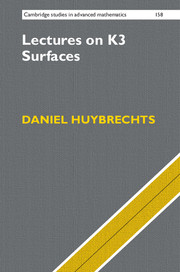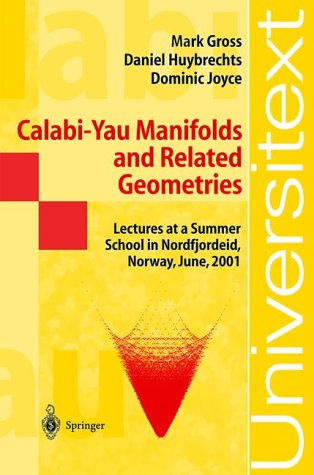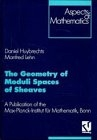### Books

1. The geometry of cubic hypersurfaces. to appear in Cambridge University Press. 456 pages. CUP allows me to keep the pdf of the final draft.
2. Lectures on K3 surfaces. Cambridge University Press. 2016. 496 pages. Old homepage with pdf of early version and an erratum is here
3. Fourier-Mukai transforms in Algebraic Geometry. Oxford Mathematical Monographs. 2006. 307 pages.
4. Complex geometry - an introduction. Springer (2004). Universitext. 309 pages.
5. (with D. Joyce, M. Gross) Calabi-Yau manifolds and related geometries. Springer (2002), 244 pages.
6. (with M. Lehn) The geometry of moduli spaces of shaves. Aspects of Mathematics E 31, Vieweg (1997), 260 pages. 2nd edition in Cambridge Mathematical Library of Cambridge Unversity Press (2010).

7.